COMPLETED EINSTEIN SPECIAL THEORY OF RELATIVITY (CSTR)

Nimit Theeraleekul B. Eng. (Communication)

(September 29, 2005, 4th. revised Aug 1, 2012)

Abstract: Einstein special theory of relativity (STR) has been criticized for lacking of its philosophical idea (mechanism which explain how the theory works), despite of their predictability and accuracy. Indeed the problems could be eliminated by using a new concept of “Vacuum Mechanics”.

Content: 1) Introduction. 2) Original of STR’s problems. 3) Newton framework & classical mechanics. 4) Einstein framework & relativistic mechanics. 5) Michelson-Morley experiment. 6) Vacuum medium privileged absolute reference frame. 7) Modification of STR. 8) Reinterpret Principle of relativity. 9) New derivation of Lorentz transformation. 10) Physical meaning of Lorentz transformation. 11) Problem with constant c in a moving inertial reference frames. 12) What is the “increasing mass”? 13) Mechanism of time dilation. 14) No length contraction is existed. 15) Relativistic Doppler Effect of acoustic wave. 16) Einstein returns to aether? 17) Conclusion & discussion. 18) References.

1) Introduction. This article is the second expanding part of the original article “Vacuum mechanics a New Approach to the Theory of Everything” (VMTE) . And because what has been talked there is rather a philosophical one, so this paper will be talked in a more scientific detail about the modification of STR. But before the modification, it is necessary to summarize main parts of VMTE which will be involved with STR as below.

1.1) In VMTE we have started with the fundamental hypothesis that “Vacuum medium is the fabric structure of vacuum space which was proved that it is not the conventional empty vacuum space; instead it is vacuum medium space! In more detail, it is the privileged absolute reference frame of a continuous isotopic homogenous medium and having a very thin constant mass density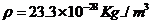which is permeable by all matters almost without any observed resistance!

Electrons and protons are tiny black holes is the consequence hypothesis, and they were proved to be the “condense” of vacuum medium. Both of them are the two elementary particles which formed to be all kinds of the material matters existed in the universe! So our universe is composed of visible material matters immerged in a huge volume of the transparent vacuum medium.

Vacuum mechanics – a new conceptual mechanics. It is the mechanism of vacuum medium, i.e. the action of vacuum medium or of condensed vacuum medium and the interaction between them, is responsible for all natural phenomena in our universe! Later we will see how this vacuum mechanics is the key for solving the philosophic problems in STR.

The next expanding article of VMTE is “Completed Maxwell electromagnetic field theory” (CEMT) , which is the improvement of Maxwell electromagnetic field theory (EMT). And because there is intimate relation between EMT and STR, so to write this article, EMT will be talked as the starting point. Also because we have to refer to it quite often, so below is a brief summary about CEMT.

1.2) In CEMT, what followed from the concept that electron and proton are tiny black holes is that when they are staying still, they will attract with the surrounding vacuum medium. And this will create the internal stress in the vacuum medium around, and which is appeared as electrostatic field. But when electron is moving, it will rotate in the direction perpendicular to the motion’s axis. Then it will create rotational stress in the surrounding vacuum medium while dragging it along, this is magnetostatic field!

We also showed that “vacuodynamics” (which is the interaction between tiny black holes and the background vacuum medium) could explain why and how electromagnetic field radiation was generated and propagate through vacuum space. That is, it is the occurrence of the dynamics action of stress-strain relations in vacuum medium. And finally we have explained that light speed is constant because it is an electromagnetic wave which is the vibration of the uniform vacuum medium!

2) Original problems of STR.     Everyone who familiar with STR would found that despite of their predictability and accuracy; it came with philosophic problems i.e. the increasing mass, length contraction and time dilation of the moving object. But No one can understand and explain clearly what the causes of these effects are? Beside the problems mentioned, the second hypothesis (of the theory) “the velocity of light is the same for any inertial observers” was also criticized to be incorrect!

Historically STR was possible because Einstein has abandoned EMT mechanical concept to make it consistent with principle of relativity. And before that, when James Clerk Maxwell had created the EMT, he also invented a mechanical model of elastic solid (aether) for its philosophical concept. Then we could understand how the electromagnetic phenomena work. But after Einstein created STR, it is no need for the aether, then people ignored Maxwell model and only the equations were used until now .

By the way, before Einstein created STR, there was a long debate about the existing of the hypothetical aether in the vacuum space. And in that day there was no obvious way for proving the existence of aether in the vacuum space. So people accepted STR which is no need the aether for its concept. But we will see later that it is starting point of all of the problems mentioned above!

To solve the problems in STR, we have to recall Newtonian classical mechanics which was said that STR was extended from it. After that, we will reconsider detail assumptions of STR to see what is not appropriate and create the problems. Finally we will improve it by using a new concept of “Vacuum mechanics”. And what we will get is a completed STR – CSTR, which could solve all the problems occurred in the conventional STR.

3) Newton framework & classical mechanics. Everyone may familiar with Newton laws of mechanics, but may be not aware with Newton’s absolute reference frame which provided as the backcloth for Newtonian classical mechanics. The concept is that empty vacuum space is the privileged absolute reference frame of the universe! Together with the concept that time is universal (i.e. time is the same everywhere) then they form to be Newton’s space-time.

One important point is that Newton first law provides the principle of relativity (and was used by Einstein as his first postulate for creating STR) which state that “The motion of bodies included in a given space are the same among themselves, whether that space is at rest or moves uniformly in forward in a straight line”. In other word, all inertial frame are equivalent!

Next according to the principle of relativity, Newton laws of mechanics could be transformed from one coordinate system (in one inertial frame) to another coordinate system (in another inertial frame) without changing their forms by using Galilean transformation. In other word, we could say that law of inertia is invariance or covariance under Galilean transformation, equation (1) – (3).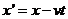……… (1),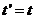……. (2),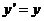,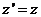……… (3).

Now let us reconsider further for testing the validity of principle of relativity mentioned above whether it is true or not. And it is easy to do it by following Richard P. Feynman (Nobel Prize winner in physics) idea  as follow; first we investigate whether Newton’s laws appear the same in the moving system as the rest one.

To do the experiment, we use light source which will generate electromagnetic waves in all direction at the same speed and equal to c, or 186,000 mile/sec. (according to Maxwell electromagnetic field theory) no matter whether the source is at rest or moving! This is analogous to the case of sound waves in which the speed of sound waves is independent of the source.

Suppose we are riding in a car moving at a speed u, and light from the rear is going past the car with speed c. Differentiating equation (1)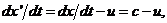……… (4).

which means that according to Galilean transformation the apparent speed of the passing light, as we measure it in the car, should not be c but should be c – u. In any case, by measuring the speed of the light going past the car, one could determine the speed of the car. And based on this general idea, several experiments were done to determine the velocity of the earth, but they all failed – they gave no velocity at all!

4) Einstein framework & relativistic mechanics. In contrast to Newton’s absolute reference frame (which provided as the backcloth for Newtonian classical mechanics); Einstein STR has no absolute reference frame (only relative is possible) in which it gives rise to relativistic mechanics, i.e. the concept of variable mass, energy and momentum (while they are constant quantities in classical mechanics).

In creating STR, Einstein has used Newton’s principle of relativity together with the postulate that speed of light is constant in any inertial frame. And then what he got is Lorentz transformation equation (1’) – (3’). (replaced Galilean transformation) which gives an invariance transformation of physics laws in transforming from one coordinate system (in one inertial frame) to another coordinate system.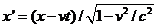(1’),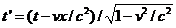... (2’),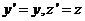… (3’).

Anyway, based on the concept that light speed is constant reference to any inertial observers (instead of reference to vacuum medium reference frame), then he had to sacrifice the conventional rigid space and universal time. That is if we calculate length and time by using Lorentz transformation, then what we will got is the contracted length and the dilated time!

Now let us return to consider the case of the moving car (mentioned above) in which the apparent speed of the passing light, as we measure it in the car, should be c – u according to equation (4). But what we have got is no relative motion between aether and the earth, and it was interpreted as light speed is constant reference to any inertial observers (Einstein’s second postulate). And of course if we use Lorentz transformation (instead of Galilean transformation) to calculate it, then we will got the result as c because it is the reverse process of derivation of Lorentz transformation in STR!

5) Michelson-Morley experiment.  Actually the experiments which were done to determine the velocity of the earth motion (mentioned above) based on the concept of the famous Michelson-Morley experiment. And it was done to determine the motion of the earth with respect to the aether (in which light was used to travel with uniform speed). The essential idea of the experiment was to compare the apparent speed of light in two different directions.

In the diagram (b), light ray from terrestrial source split into two parts at the silvered glass P. One of the two light parts travel via path L1 to one of the two mirrors which placed at right angle (with equal distance from P) and then reflect back to reunite with the other which travel via path L2 at the observer. The two parts light rays will interfere forming interference fringes

(which could be observed via a telescope F) if the light ray which travels via path L2 takes lesser time than the other one which travel via path L1.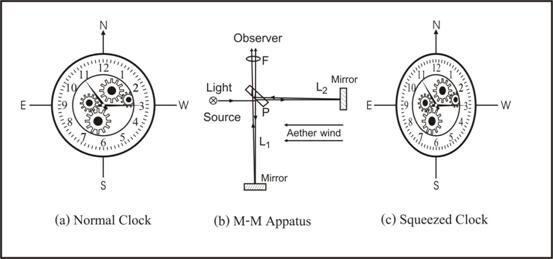Figure 1 M–M experiment vs. Magic clock.

Everyone know that the experiment result give no sign of interference fringes, and which means that travelling times of the two light rays is the same. From the result of the experiment, which show that there is no relative motion between the earth and the aether, and then it was interpreted that no aether is existed!

Anyway that the null result of the experiment may also due to the dragged along of the aether! And we could explain the reason of the appearance of stars aberration, that is it is because of the large distance between stars and the earth while the dragged along of the aether is a small portion as noted by Michelson himself . Also the earth rotational velocity is small compare to light, so the dragged effect is so small and could be neglected.

It is interesting to note that mainstream physicists believe that the null result of the experiment was due to length contraction of the instrument’s arm L1 (according to the conventional interpretation using STR), rather than the aether drag. And in order to visualize and understand what happen with the experiment apparatus; we will use an analogy of the magic clock shown in (c).

In detail, Michelson-Morley experiment was done on a circular rotating platform lay horizontally. Now suppose we place a normal clock on the platform, then the clock’s shape would be squeezed and having a distorted shape as shown in diagram (c). The reason is because there is a length contraction of the clock in the earth rotation direction!

Remember that the squeezed clocks were observed by someone who stays for example, in the sun rest reference frame. For us who stay on the moving earth with the clock, what we sew is the normal (shape) clock! This is not because the distortion is too small to be noticed, but it is because the ruler is also distorted in the same scale. So it seems that we could never seen the distort part of the clock, and indeed we are facing with a magic clock!

In conclusion, up to now we can say that there is no experiment (except the Michelson-Morley experiment) which was made and shown clearly that there is no relative motion between the earth and the aether. So what we need more now, is to do an additional experiment test on other moving frame (besides the moving earth) such as in the space shuttle or space station (which will be explain next) , and then it will be the final judgment!

6) Vacuum medium privileged absolute reference frame. Nowadays we will not argue further about the result of Michelson-Morley experiment, instead we will prove that the aether (which we called vacuum medium), is obviously existed by using a simple scientific experiment. (Detail could be found in VMTE .)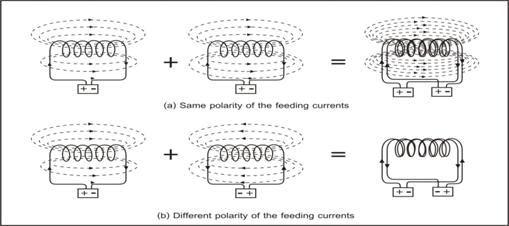Figure 2 Two-solenoid experiment

In the diagram (a), sum of the created magnetic field is double, while in (b) the sum is zero. This is what it should be according to electromagnetic field theory, but the problem is where the created magnetic field energy was gone? We know that energy cannot disappear because it will violate the principle of conservation of energy! So the only sensible answer is that the magnetic energy was used to cancel the opposite rotational stress in the physical vacuum medium space! This is an obvious indirect prove for the existence of the aether, isn’t it?

As mentioned early (in the introduction) that vacuum medium is the fabric structure of vacuum space of our universe. In more detail, it is a continuous isotopic homogenous medium and having a peculiar mechanical property; while it is very thin in constant mass densitywhich is permeable by all matters almost without any observed resistance, it has a very large elastic coefficient and sensitive to shear force or rotational force!

To imagine how the vacuum medium look likes, it is instructive to compare it with our breathing air. What similar between air and vacuum medium is that both of them are transparent. While it seems that air’s mass density is very dilute (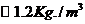), but we could see from above paragraph that vacuum medium mass density is much more dilute. This is the reason why it is very difficult to detect its existence directly.

Finally, we often heard that vacuum medium should be very rigid for creating such a huge speed c of light! This idea comes from the concept that sound speed in rigid medium such as steel which is greater than the non-rigid medium such as air, for example. But this is not quite right; the better method is to compare the same kind of medium such as normal air and hydrogen. For air’s mass density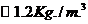which has sound speed 331m/s, while hydrogen mass density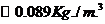has sound speed 1284m/s. The reason is because mass density of hydrogen is lower than the air (assumed both have the same modulus), so its sound speed is higher than air according to wave speed formula which equal to square root of modulus over density.

7) Reinterpret principle of relativity. Once we have vacuum medium space as privileged absolute reference frame, we cannot use the conventional interpretation of the relativity principle anymore. The reason is because we can distinguish a moving frame from a rest privileged absolute reference frame, i.e. physics laws is not the same for all inertial reference frame!

In order to keep the concept that physics laws is the same for all inertial reference frames (whether it is a moving or rest frame), we have to reinterpret the old relativity principle (which is valid only for the concept of empty vacuum space) to a new realistic physical space of vacuum medium as “physics is the equivalent referenced to vacuum medium space”!

Now let us make a simple experiment involved light signal in a moving spaceship which passing through vacuum medium (both outside and inside spaceship). Then we would able to measure the speed of the moving ship. And this means that we could violate the old principle of relativity, so let us see how it could be done, and why it was not be found before.

Reference to the method used to find “the relativity of time and length” in reference . Two measurements on the moving spaceship were done. One measurement is used to find the traveling time for light to go and back in the direction transverse to the motion, another one is used to find the traveling time for light to go and back in the direction parallel to the motion.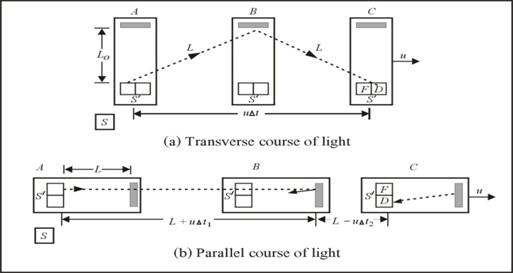Figure 3 Measurement of light in a spaceship.

According to the fig.3 (b) above, let c = velocity of light in vacuum medium reference frame.

Time for light moving round-trip up and down in stationary spaceship,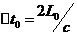…… (1).

Time for light moving round-trip up and down in moving spaceship,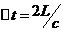……….... (2).

From (1) and (2) we get, the increasing time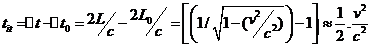…….….. (3).

Now we will measure the increasing traveling time of light in the direction parallel to the motion of the spaceship, and according to the fig.3 (a) above;

Time for light moving round-trip back and fore in stationary spaceship,…. (4)

Light’s time moving round-trip back & fore in moving spaceship,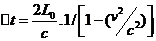(5)

From (4) and (5) we get, the increasing time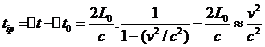...(6)

If speed of spaceship (or space station) = 30 km/sec.

Then from (6), the increasing traveling time of light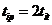= 0.0000006 nanosec.

From the above examples we found that even for the fastest man made vehicle, we still cannot directly measure the increasing time of light with present technology (less than order of nanosecond). This show that how small affect is occurred for a moving object and this is the reason why we cannot observe any error for the principle of relativity!

Anyway if we want to measure the increasing time of light, we have to use an indirect measurement (in spaceship) such as the famous Michelson-Morley experiment or Trouton-Noble equipment , and then calculate back to the velocity of the spaceship.

[Note that for the concept of light speed is constant reference to spaceship (as was done in the reference), what they got from equation (3) and (4) is as (7)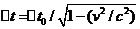…… (7)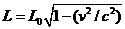….. (8)

This was interpreted as “time dilation”. And also from equation (3) and (4) they got “length contraction” on the assumption that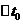is the same whether the spaceship is moving or not as in (8).]

In conclusion, up to now we can say that nowadays there is no experiment test for proving the validity of the principle of relativity. And of course the final judgment is based on the result of the proposed experiment in the space shuttle or space station mentioned above!

8) Modification of STR. Now, we are ready to improve STR by modify it from a non aether-based theory (which based on empty vacuum space concept) to an aether-based theory which correspond to the concept of vacuum medium space. Anyway before doing a modification, let us start with summarize about the idea in conventional STR.

First, the conventional STR was start from the concept that Newton classical mechanics is invariance in Newtonian absolute empty space and universal time, i.e. the principle of relativity. Next in order to extend the principle to cover the invariance of electrodynamics in Maxwell electromagnetic field theory (i.e. to make all physical laws to be the same referenced to any inertial frames - the first postulate of Einstein), then Einstein has invented the second postulates - velocity of light is the same for observer in all inertial frames.

Now to modify STR and what we will do first is to change the first postulate from “Physics are equivalent referenced to any inertial frames” to “Physics is equivalent referenced to the rest frame of vacuum medium space”! It is no need to change the second postulate from “the velocity of light is the same for any observers” to “velocity of light is the same referenced to vacuum medium rest frame”. The reason is because the velocity of any natural waves in any homogeneous medium is depending on it property and always constant!

9) New derivation of Lorentz transformations. Now under our new concept of vacuum medium space, we will derive Lorentz transformation equations by using k-calculus (as it was done in the reference ) as the guideline. Together with some of the assumptions and their interpretation were changed as below.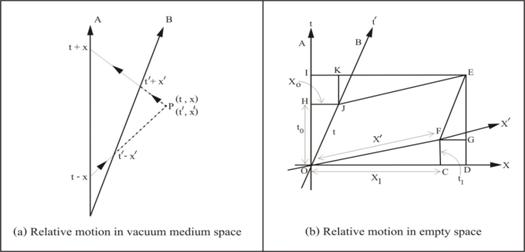Figure 4 Relative motion between A and B in space-time

Reference to diagram in figure 4 (a), A and B are the world–lines of two relative motion observers. A is at rest to vacuum medium, while B is moving with constant velocity v. Let P is an event at a coordinate (t, x) relative to A and (t’, x’) relative to B. When A send out a light ray (c = 1) at time t-x to illuminate P at time t and also receive the reflected ray back at time t+x.

Similar remarks apply to B, where we use primed quantity. Assuming A and B synchronize their clock when they met. Then by some arrangement and using the definition of k-factor, we get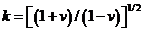……….. (9).

Combining to the space-time coordinate relation,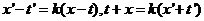…. (10).

Then we get Lorentz transformations as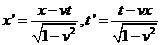….. (11).

Or in the form including the velocity of light c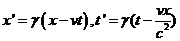…… (12).   [Where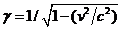]

Note that for derivation of the Lorentz transformations by using the concept of the old STR (constant c in a moving inertial frame)  as shown in figure 4 (b), it has to involving with time dilation and length contraction. And below is a brief summary of the derivation.

From the diagram we get

Time dilation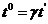…. (13), Length contraction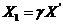…… (14).

Then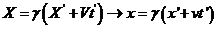…… (15).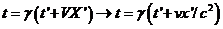(16), together with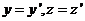… (17).

Equations (15), (16) and (17) are Lorentz transformations!

Comparing the Lorentz transformations derivation between the new concept (constant c referenced to vacuum medium) in fig.4 (a) and the old concept (constant c referenced to an inertial reference frame) in fig.4 (b), we would found that the former is easy and understandable. While the latter is so complicate, we have to add the assumptions time dilation and length contraction in order to keep the constant speed of c referenced to the moving frame. How could we understand why it is so? And for the former, we could make measurements of the graphic in diagram to get the Lorentz transformations directly! Also we could see clearly that light speed c referenced to rest frame by making an angle 45 degrees with the axes, but not to line B.

Up to now, by using the new concept of vacuum medium space we have derived the same Lorentz transformation equations as Einstein had done! And under this new concept we will see that it is possible to explain and solve the problems existed in the conventional STR. So it should be a complete STR as desired! Below are examples of explanations and solutions to some crucial problems in STR.

10) Physical meaning of Lorentz transformation. Everyone who familiar with Lorentz transformation would found that there is no physical meaning about them. When it was derived by Einstein via using concept “the velocity of light is the same for any observers” together with relativity principle, then what we got is only mathematical formulas. We have no idea why it is so!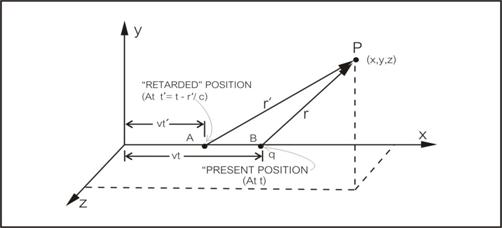Figure 5 A moving charge’s potential with constant velocity.

Now let us see the transformations which were derived by Lorentz himself . According to the diagram in fig. 5 we will find the potential at point P (x, y, z) due to a moving charge q with constant speed v. If t = 0 is the moment when the charge q is at origin, at the time t the charge is at x = vt, y = z = 0. And we know that its position at the retarded time is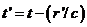, ………… (18).

From Lienard-Wiechert potential, the potential at P is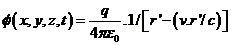……. (19).

After solving to find the potential in the present coordinate, we get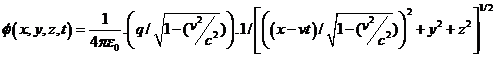… (20).

If the charge is rest at the origin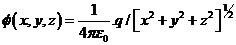………. (21)

Finally by compare the potential from the moving coordinate system (20) to the rest frame (21), it appear that the coordinate should be transformed by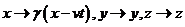[Where]

This is Lorentz transformation which Lorentz discovered it. Anyway, we still could not get any physical meaning from this derivation too!

In order to find physical meaning of the Lorentz transformation from the derivation above, we will use the concept of vacuum mechanics by looking in detail at the interaction between the moving charge and vacuum medium environment as follow.

a) According to Galilean transformation, once when the charge was placed at the origin O, its potential in equation (21) will spread out and reach point P (or anywhere else) at the same instantaneous time! But in reality the electric potential from the charge q at O needs “time” to propagate (through vacuum medium) to reach P (with light speed c) after the instant which it was placed.

b) At any instant after time t = 0, while the charge is moving from the origin with speed v < c, at the same time its spreading potential will lead ahead it with speed c.

c) When the moving charge is reaching B, its potential from point A is also reaching P, not the potential from point B which we thought by using common sense! And this is different from Galilean transformation (with the concept of infinite speed of light) which we will found that at the same instant of the moving charge reaches B, its potential will also reach point P.

d) The time at position A, and the distance between the position A and the position P (in x direction) are the time t’ and the distance x’ which appeared in Lorentz transformation (12). The retarded time t’ is existed because there is a relative motion between the moving charge and the speed of light through vacuum medium!

e) So the “distance” and “time” between the interaction of  the moving charge q and the test charge at P which was measured using light speed c (via vacuum medium) is the relative “distance” and “time” occurred via Lorentz transformation. In contrast with the “distance” in Galilean transformation this was measured by using infinite light speed (via empty vacuum). And we cannot measure “timebecause of infinite light speed, i.e. time is universal (simultaneous action at a distance) according to Galilean transformation!

In conclusion, the physical meaning of Lorentz transformation is “the transformation which gives the relative apparent distance and time between two relative moving inertial frames measured by using light speed c via vacuum medium space (while Galilean transformation deal with imaginary infinite light speed via empty vacuum space)!

11) Problem with constant of c in a moving inertial reference frames. To see the problem (as mentioned early) clearly, let us see an illustration that was done by Albert Einstein , which shows “speed of light is the same c for observers in any inertial reference frame (resting or moving)” in the diagram of fig. 6 below.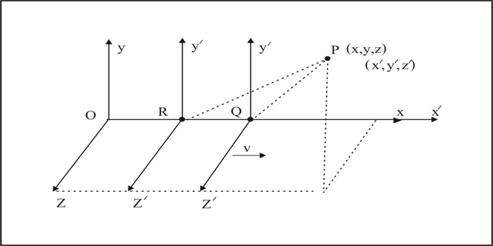Figure 6 Two observers in two relative reference frames.

A light signal is sent along the positive x-axis of a stationary frame S, and this light signal will propagate in according with the equation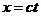.

Now if we substitute x for the value ct in equation (12) of Lorentz transformation (section 9), then what was got is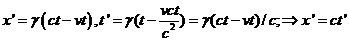.

Finally Einstein concluded that light signal is also c in the moving inertial frame S’!

But, let us interpret its physical meaning by comparing to the concept of electric potential propagate from the moving charge in fig. 5 section 10. Based on the concept of vacuum medium space, the potential (from the charge) propagates with light speed c referenced to vacuum medium. And we could see that R is the position at retarded time t’, while the appearance Q (according to Galilean transformations) is where in the old concept of STR thought it is. And if we insist that this is the position (Q) where the light signal was sent to P, then we have to suffer from time dilation and length contraction! That is because electromagnetic field propagates with light speed c independent of the motion of the source; it is analogous to the speed of sound wave [4’].

[Remember that photons (lights) are wave packets which were created during the excited electrons in atoms (of the source) rapidly changing their states and then disturbing the surrounding vacuum medium. And once after the vacuum medium was disturbed, photons’ speed is the speed of the waves in vacuum medium and not depends on the moving source any more.]

Then do we say that Einstein wrong? The answer is no, not exactly! The point is that he tried to keep the validity of the principle of relativity. So in order to do that, he has to sacrificed space and time. And what followed is the concept of length contraction and time dilation!  Now someone will further ask why in the conventional derivation with old concept (constant c reference to a moving inertial reference frame) we still got the correct transformations. The answer is that there is something which was overlook!

They said that at time = 0 (t=t’=0 and x=x’=0) both K and K’ coincide, then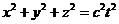and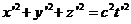which is okay (because at time t=0, S’ is in the same situation as the resting S). But when S’ is moving thenmay be right or wrong, we could not be sure whether light speed c is reference to S or S’. That is the reason why Einstein had to add the second postulate to confirm that the first postulate was fulfilled!

Lastly, we come to the crucial point that how could we sure what reference is the constancy of c refers to? Up to now there is no such experiment was made, except the Michelson- Morley famous experiment. But as we knew that the null result of the experiment may also due to the dragged along of vacuum medium, which we have discussed early. So what we need more is to do an additional experiment test on other moving frame besides the moving earth for the final judgment!

12) What is the “increasing mass”? As mentioned early that we do not know the cause which makes a moving object increase its mass. Armed with vacuum mechanics concept, it is easy to understand what it is, why and how it does, as follow.

Consider again the Lienard-Wiechert potential of a “moving” charge as in the equation (19) and rewrite in the form (22) below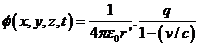……. (22),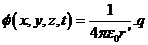…… (23).

When we compare it to a “static” electric potential of a stationary charge (23) above, we would found that the moving charge appeared to “increase its charge” by a factor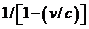which is equivalent to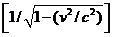in its present position in (23). (Please see detail in the reference .)

And we known that gravity has the same attraction rule as Coulomb law in electricity, if we replace the charge q with a mass then we would found that its gravitation potential is the same form as electric potential above. So the “static” gravitational potential of a stationary mass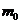is as (24), while the “moving” gravitational potential of the moving mass is as (25) below.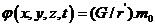…. (24),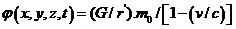….. (25).

Then we could found the gravitational potential at P in the present coordinate, in the same way as the moving charge, and we get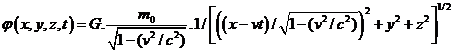….. (26).

Lastly, we could see that the moving mass appeared to increase its mass by a factoras in (25) or equivalent toas in (26)  ( in the same way as a “moving” charge do as showed above)!

Now we will consider what the “increasing mass” is. From the gravitational potential in equation (24) and (26) we could change it to the “action force F acts at a unit of test mass at P by squaring the distance in the dominator as in equation (27) and (28) below.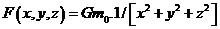…………………………………….. (27).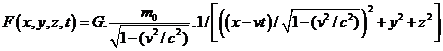….. (28).

Next, in classical mechanics where Newton gravitational law and Galilean transformation were used for equation (28) then it will change to equation (29) below.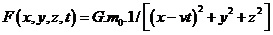…………. (29).

[Note that the gamma factor of the x – component distance in (28) means that actually the moving mass at retard position A which sent the force to P, not the moving mass at B does! But in (29), the position A is the same position as B because the velocity of force is infinite (force need no time for traveling). Here, the situation is the same way as the potential of the moving charge does as explained in the former section.]

Finally, when we compare equation (28) to equation (29) we could found that the moving mass seems to “increase its intrinsic mass” without any cause! But if we accept that the mass is immerged in vacuum medium, then we could understand that the “increasing of mass” represent the “additional mass” due to the “resistance of vacuum medium” to the moving mass!

By the way, to visualize how the inertial resistance of vacuum medium is the cause of the increasing mass of the moving object, it is easy to use the analogy that the dragging force of the surrounding air which act on a moving car (a flying plane etc.) due to the existing of the earth atmosphere.

In our daily life, from our driving car experience, in which we will found that at low speed the fuel consumption rate, is less than at high speed, the faster car - the higher consumption rate. The reason is because of air’s resistance, the faster speed - the higher resistance. In another view, we can equally say that the faster moving car means the greater increasing mass of the car!

Lastly let us suppose that we are driving in an air free tunnel without air’s resistance, then we would found that the fuel consumption rate is constant at any speed and which also means that the moving car’s mass is constant too! This is the concept of Galilean transformation which works under the concept of Newton absolute empty space!

13) Mechanism of time dilation.  According to the conventional STR, space-time could be distorted, i.e. time and space could be varied. In contrast to our new concept of CSTR, space and time cannot be varied! Anyway, we have seen some evidences which were thought as real time dilation, so we will see what really it is!

First let us see the slowing of disintegration’s time from muons which were used as the evidence of time dilation. Here we can explain its mechanism without using the conventional concept of time dilation. Instead it is obvious and easy to use the concept of increasing mass (both from the old STR or our new CSTR) for the explanation!

We know that those muons which come from the cosmic ray traveling to the earth with the great speed, so the increasing of its mass is the answer for the slowing of disintegration’s time. This is because more mass need more disintegration’s time, which is more rational than the time dilation! (Note also that both the formulas of the increasing mass and time dilation have the same form.)

Next, we could also use the concept of the increasing of mass of the oscillating atoms in an atomic clock for explaining the slowing time when it was carried in a jet plane around the world. This is because of the slower oscillation of the atoms in the moving atomic clock due to its increasing mass! It is not the “true” time dilation measured by the moving clock, but it is the slowing of the mechanism of the clock!

In conclusion time dilation is an apparent time dilation which occurred by measurement between any two initial frames moving relative to each other, i.e. it is not a true natural time dilation! Anyway, even time dilation is a relative time, but because the “time” was measured by a moving clock (in the moving frame), so it runs slower due to the dynamic resistance of vacuum medium - the mechanism of time dilation!

By the way, in order to prevent misunderstanding the meaning of the conventional time dilation (i.e. the real time dilation), it is better to call it (the conventional time dilation) as the “relative time”, while the time dilation due to slowing of the moving clock should be called as “relativistic time”!

14) No length contraction is existed. Among the other relativistic effects in STR, length contraction is one which has no experiment evidence to confirm it! This is because there is no “pure” length in nature that can be measured while there are being hurled about at relativistic velocities. It is the same reason as that there is no experiment evidence to confirm the relativity of simultaneous [7’].

Actually, conventional length contraction is relative length measured between any two initial frames moving relative to each other; it is just an apparent length contraction not a real physical length contraction! As mentioned early in section 6 that actually the conventional length contraction was arisen because it follows the conventional time dilation concept. And in order to prevent misunderstanding of its meaning (such as using it as the reduction of a volume and get the increasing in density), it should be called as relative length!

By the way, as we have point out that time dilation and length contractions occur because Einstein used the concept that “light speed is constant reference to the observer”, instead of “reference to vacuum medium”. Now, according to the concept of constant speed of sound wave in air, which was used in deriving relativistic Doppler Effect formula ( see detail in next section), it is analogous to constant c in vacuum medium’s concept. And there is no length contraction and time dilation in both cases. So this could give us confidence that length contraction was misinterpreted!

15) Relativistic Doppler Effect of acoustic wave. Armed with our new aether based CSTR, we could explain some unsolved problems which could not be done by the conventional (non-aether based) STR. Here we will talk about the misunderstanding of Doppler Effect for sound wave as an example.

Conventionally when we deal with Doppler Effect, we would found that it is so different between   and light wave. The reason is that we accept that sound wave needs a medium (air) for travelling, while light wave need no any medium for propagation! Then it was concluded that there is no Relativistic Doppler Effect for sound wave! Now under our new concept of the existing of vacuum medium space, the situation is changed, so we could derive relativistic Doppler Effect formula for sound wave in the same way as of the light one.

First, we will use k-calculus to derive Lorentz transformation for air medium. So we have to use sound radar (radar using Doppler-shift sound wave signal instead of light signal) for measuring. The method is the same way as was done in the diagram of figure 4(a). But now the diagram is air’s space-time not vacuum medium space-time! Then we will get the Lorentz transformations for air in the same way as in (11) section 6 except c was replaced by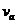the velocity of sound wave in air, that is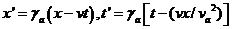….. (30).      [Where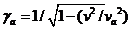.]

Next is to derive classical Doppler Effect for sound wave in air, and below is the summary of the conventional derivation of sound wave in the reference [8’].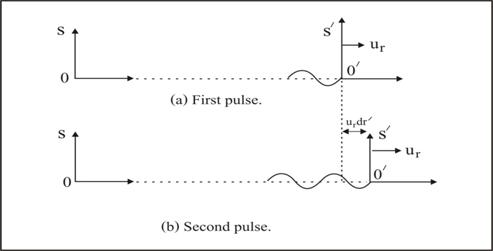Figure 7 Doppler Effect of sound wave.

According to the diagram in fig. 7, an emitting light source with wavelength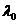is fixed in the moving reference frame S’. An observer is staying in the rest reference frame S. Two successive light pulses are emitted from S’ (which is moving with a radial velocity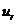) with a time difference by dt’ as measure by S’. The distance that these two pulses have to travel with speed c will differ by an amount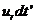, and arrive at S with a time different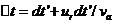…(31)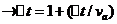….(32). And because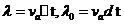,

then we will get the classical Doppler formula for sound wave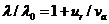… (33).

(Where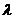= received wavelength of the rest observer,= emitting wavelength of the moving source,= radial speed of the moving source,= speed of sound wave in air medium.)

Finally, because equation (33) was derived from the concept of Galilean transformations (imaginary space-time with infinite sound speed), now we are dealing with air’s physical space-time with finite sound speed, i.e. Lorentz space–time using sound speed as measuring tool. So we have to include its effect – the gamma factor of Lorentz transformation for air medium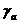in (31), that is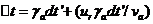. Then the final result is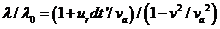.

(Note that in conventional way, they corrected it with what they called time dilation.)

And if the moving source is purely radial (= v), then what we got is the relativistic Doppler Effect for sound wave as desired in (34) below.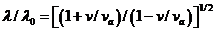……… (34).

16) Einstein returns to aether? In recent years there is an unfamiliar of Einstein’s quotation “ETHER AND THE THEORY OF RELATIVITY” which was addressed on May 5th, 1920 at University of Leyden . And here is the conclusion in the final paragraph;

“Recapitulation, we may say that according to the general theory of relativity space is endowed with physical quality; in this sense, therefore, there exists an ether. According to the general theory of relativity without ether is unthinkable; for in such space there not only would be no propagation of light, but also no possibility of existence for standards of space and time (measure-rod and clocks), nor therefore any space-time intervals in the physical sense. But this ether may not be thought of as endowed with the quality characteristic of ponderable media, as consisting of parts which may be tracked through time. The idea of motion may not be applied to it.”

As mentioned in the above address it seems that Einstein has change his mind about the existence of the aether, which is contrast to what we have learnt and understood that the conventional STR (that he invent it in 1905) had rule out the existence of aether! This was point out by Frank Wilczek, a Nobel Prize winner in physics 2004 in his book “The Lightness of Being (Mass, Ether, and the Unification of forces)” . Anyway, there is no detail about Einstein’s aether, and it seems this is not the same thing as the aether in 19th century physics!

For me (the author), it seems that the important point which Einstein wanted to do in the address is to add some physical thing for acting as the mechanism for light and gravity in order to fulfill his relativity theory. Anyway, he did not want the old aether, but rather be something which looks like the aether! The reason is because space which filled with the aether will destroy the principle of relativity, i.e. all physical laws will not invariance. And may be because there is no obvious prove for the existence of aether at that time, so it is not so much trouble for Einstein to accept his new kind of aether.

Nowadays, we have obviously proved for the existence of vacuum medium as a new aether, and after reinterpretation the principle of relativity, then what we got is the Lorentz transformation in our improved STR (i.e. CSTR) which makes physics is invariance under Lorentz transformation!

17) Conclusions & discussion. Finally we have seen how the conventional STR was improved by adding it with a rational philosophical idea; the mechanism for relativistic mechanics i.e. the mechanism of vacuum medium (or vacuum mechanics)! And we have seen that lacking of the mentioned mechanism had lead to some crazy thing (due to misinterpretation) such as the increasing of mass, time dilation and length contraction.

Anyway, the mentioned problem was not Einstein’s fault; instead it is the best way which he could do to get the great STR just by using his genius. He had avoid the conflict about the aether concept (in his time) by ignore it. For the author opinion may be Einstein himself was not so satisfied with it, but this is the best choice to do if we choose not to use something such as aether!

Actually may be there is no need to modify the conventional STR if we want to use it separately from other theory. Anyway, one important consequence of this improved theory (CSTR) is that it will lead to a more complete Einstein general theory of relativity (CGTR) because GTR is the extension of STR. Then this could provide a better way for GTR to be able to combine with quantum mechanics (QMT) in order to get a successful the theory of everything!

By the way, we could see that what was written in this paper is a short one, and it is not fully mathematical rigor. What we have done is just enough to give the new idea to the readers with some confidences. So what was left here should be the work of people who are involved in the matter to complete it for the virtue of academic merit and the progress of our world’s knowledge!

18) References.

(Precaution; Several text books with different authors were used as the reference and each author used different pattern and different notation for the same formula. Here in this paper, the author has made some change of the original pattern and notation for the readers convenient, so please be careful!)

 Nimit Theeraleekul, “Vacuum Mechanics a New Approach to the Theory of Everything”

present in www.vacuum-mechanics.com.

 Nimit Theeraleekul, “Completed Maxwell electromagnetic field theory” now presenting in

 James Blake Westgard, “Electrodynamics: A Concise Introduction” p. 90-95.

 Richard P. Feynman “SIX NOT-SO-EASY PIECES” First printing, March 1997 p. 50-53.

For [4’] p. 63.

 Sir Edmund Whittaker F. R. S., “A History of the Theories of Aether and Electricity” Vol.1,

p.391.

 D. Halliday, R. Resnick, and K. Krane, “Physics” Vol. 1 Extended Version Fourth Edition

p. 470-472. For [6’] p.482-483.

 Richard A. Mould “Basic Relativity” Corrected second printing 1995, p.13-16, for [7’] p.82

 Ray d’Inverno “Introducing Einstein’s Relativity” Reprinted 1993 p. 25. For [8’] p.39-40.

 George F.R.Ellis and Mauro Carfora “Flat and Curved Space -Times” Second edition

2000 p. 122-127.

 Richard P. Feynman “The Feynman Lecture on Physics” Volume 2 p. 21-12 – 21-13.

 Albert Einstein “Relativity (The special and general relativity)” p. 36-39.

 Frank Wilczek, “The Lightness of Being” chapter 8 - The Grid (Persistence of Ether)

p. 73 - 111.